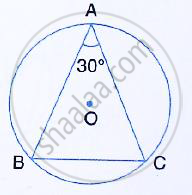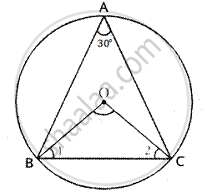Share

# In the Given Figure, Abc is a Triangle in Which ∠Bac = 30°. Show that Bc is Equal to the Radius of the Circumcircle of the Triangle Abc, Whose Centre is O. - Mathematics

Course

#### Question

In the given figure, ABC is a triangle in which ∠BAC = 30°. Show that BC is equal to the radius of the circumcircle of the triangle ABC, whose centre is O.#### SolutionGiven – In the figure ABC is a triangle in which ∠A = 30°
To prove – BC is the radius of circumcircle of ∆ABC whose centre is O.
Construction – join OB and OC.
Proof :
∠BOC = 2∠BAC = 2 × 30°= 60°
Now in ∆OBC,

OB = OC         [Radii of the same circle]

∠OBC = ∠OCB
But, in ΔBOC,
∠OBC + ∠OCB + ∠BOC = 180°     [Angles of a triangle]
⇒ ∠OBC + ∠OBC + 60° = 180°
⇒ 2∠OBC + 60° = 180°
⇒ 2OBC = 180° - 60°
⇒ 2∠OBC = 120°
⇒ OBC = (120°)/2 = 60°
⇒ ∠OBC = ∠OCB = ∠BOC = 60°
⇒ ΔBOC is an equilateral triangle
⇒ BC = OB = OC
But, OB and OC are the radii of the circum – circle
∴ BC is also the radius of the circum – circle

Is there an error in this question or solution?